MTG Vedic Mathematics combo Vol-1 to 7

693.00

Vedic Mathematics is an ancient mathematics skill to do sharp calculations. The steps illustrated in these books enable students to understand the Vedic Mathematics sutras in simple language. The workbook format of the book helps students to do practice in the space provided. Students will be able to master calculation in all mathematical operations.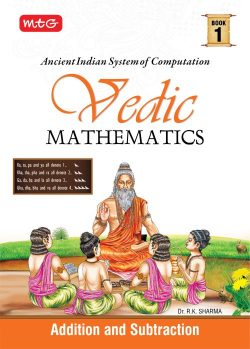1 × MTG Vedic Mathematics Vol-1 : Addition and Subtraction

591 in stock

99.00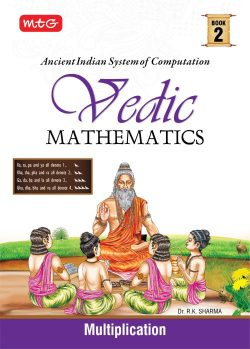1 × MTG Vedic Mathematics Vol-2 : Multiplication

592 in stock

99.00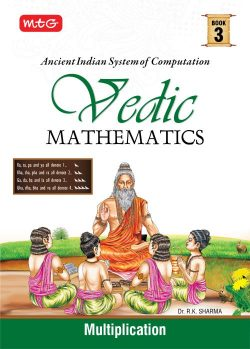1 × MTG Vedic Mathematics Vol-3 : Multiplication

592 in stock

99.00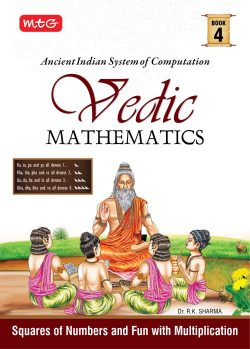1 × MTG Vedic Mathematics Vol-4 : Squares of Numbers and Fun with Multiplication

592 in stock

99.00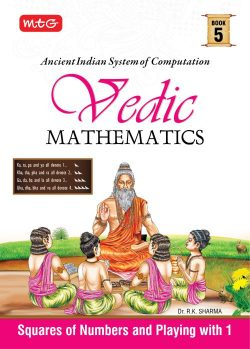1 × MTG Vedic Mathematics Vol-5 : Squares of Numbers and Playing with 1

593 in stock

99.001 × MTG Vedic Mathematics Vol-6 : Multiplication

593 in stock

99.00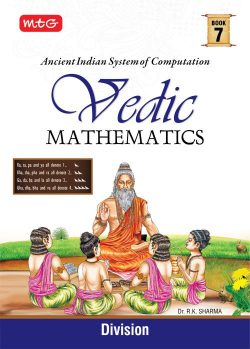1 × MTG Vedic Mathematics Vol-7 : Division

593 in stock

99.00

591 in stock

Qty:Add to WishlistVedic Maths Course is for students, teachers, mentors, homemakers and even businessmen for doing calculations more efficiently. With the help of Vedic Maths, one can perform calculations 10-15 times faster than the conventional maths methods. The current series is based on the natural way of learning which helps to learn and perform calculations at a much faster rate with an efficiency that can save time in solving mathematical problems. It creates interest in the evolution of mathematical computation in a more integrated and efficient manner. Calculations are carried out mentally which means students can invent their own methods through Vedic Sutras. In competitive exams like JEE, SAT, CAT, MAT, GRE, etc. where speed and accuracy play a vital role, learning Vedic Maths can prove to be very beneficial.

Combo Contains: 7 books
MTG Vedic Mathematics Vol – 1: Addition and Subtraction
MTG Vedic Mathematics Vol – 2: Multiplication
MTG Vedic Mathematics Vol – 3: Multiplication
MTG Vedic Mathematics Vol – 4: Squares of Numbers and Fun with Multiplication
MTG Vedic Mathematics Vol – 5: Squares of Numbers and Playing with 1
MTG Vedic Mathematics Vol – 6: Multiplication
MTG Vedic Mathematics Vol – 7: Division

Condition New Class 2 Olympiads Science

Reviews

There are no reviews yet.